Related Articles
Biconnected graph
• Difficulty Level : Hard
• Last Updated : 27 Oct, 2020

An undirected graph is called Biconnected if there are two vertex-disjoint paths between any two vertices. In a Biconnected Graph, there is a simple cycle through any two vertices.

By convention, two nodes connected by an edge form a biconnected graph, but this does not verify the above properties. For a graph with more than two vertices, the above properties must be there for it to be Biconnected.
Or in other words:
A graph is said to be Biconnected if:
1) It is connected, i.e. it is possible to reach every vertex from every other vertex, by a simple path.
2) Even after removing any vertex the graph remains connected.

Following are some examples.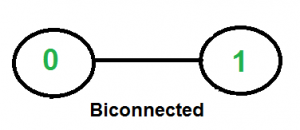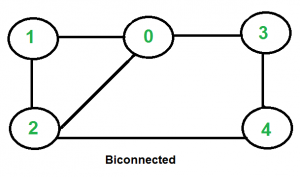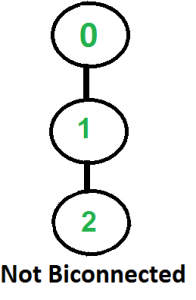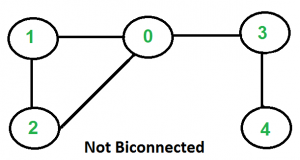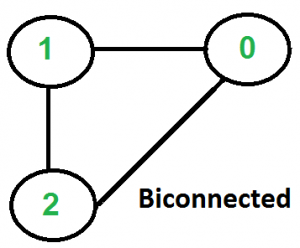See this for more examples.
How to find if a given graph is Biconnected or not?

A connected graph is Biconnected if it is connected and doesn’t have any Articulation Point. We mainly need to check two things in a graph.
1) The graph is connected.
2) There is not articulation point in graph.

We start from any vertex and do DFS traversal. In DFS traversal, we check if there is any articulation point. If we don’t find any articulation point, then the graph is Biconnected. Finally, we need to check whether all vertices were reachable in DFS or not. If all vertices were not reachable, then the graph is not even connected.

Following is the implementation of above approach.

## C++

 `// A C++ program to find if a given undirected graph is``// biconnected``#include``#include ``#define NIL -1``using` `namespace` `std;` `// A class that represents an undirected graph``class` `Graph``{``    ``int` `V;    ``// No. of vertices``    ``list<``int``> *adj;    ``// A dynamic array of adjacency lists``    ``bool` `isBCUtil(``int` `v, ``bool` `visited[], ``int` `disc[], ``int` `low[],``                 ``int` `parent[]);``public``:``    ``Graph(``int` `V);   ``// Constructor``    ``void` `addEdge(``int` `v, ``int` `w); ``// to add an edge to graph``    ``bool` `isBC();    ``// returns true if graph is Biconnected``};` `Graph::Graph(``int` `V)``{``    ``this``->V = V;``    ``adj = ``new` `list<``int``>[V];``}` `void` `Graph::addEdge(``int` `v, ``int` `w)``{``    ``adj[v].push_back(w);``    ``adj[w].push_back(v);  ``// Note: the graph is undirected``}` `// A recursive function that returns true if there is an articulation``// point in given graph, otherwise returns false.``// This function is almost same as isAPUtil() here ( http://goo.gl/Me9Fw )``// u --> The vertex to be visited next``// visited[] --> keeps tract of visited vertices``// disc[] --> Stores discovery times of visited vertices``// parent[] --> Stores parent vertices in DFS tree``bool` `Graph::isBCUtil(``int` `u, ``bool` `visited[], ``int` `disc[],``int` `low[],``int` `parent[])``{``    ``// A static variable is used for simplicity, we can avoid use of static``    ``// variable by passing a pointer.``    ``static` `int` `time` `= 0;` `    ``// Count of children in DFS Tree``    ``int` `children = 0;` `    ``// Mark the current node as visited``    ``visited[u] = ``true``;` `    ``// Initialize discovery time and low value``    ``disc[u] = low[u] = ++``time``;` `    ``// Go through all vertices aadjacent to this``    ``list<``int``>::iterator i;``    ``for` `(i = adj[u].begin(); i != adj[u].end(); ++i)``    ``{``        ``int` `v = *i;  ``// v is current adjacent of u` `        ``// If v is not visited yet, then make it a child of u``        ``// in DFS tree and recur for it``        ``if` `(!visited[v])``        ``{``            ``children++;``            ``parent[v] = u;` `            ``// check if subgraph rooted with v has an articulation point``            ``if` `(isBCUtil(v, visited, disc, low, parent))``               ``return` `true``;` `            ``// Check if the subtree rooted with v has a connection to``            ``// one of the ancestors of u``            ``low[u]  = min(low[u], low[v]);` `            ``// u is an articulation point in following cases` `            ``// (1) u is root of DFS tree and has two or more chilren.``            ``if` `(parent[u] == NIL && children > 1)``               ``return` `true``;` `            ``// (2) If u is not root and low value of one of its child is``            ``// more than discovery value of u.``            ``if` `(parent[u] != NIL && low[v] >= disc[u])``               ``return` `true``;``        ``}` `        ``// Update low value of u for parent function calls.``        ``else` `if` `(v != parent[u])``            ``low[u]  = min(low[u], disc[v]);``    ``}``    ``return` `false``;``}` `// The main function that returns true if graph is Biconnected,``// otherwise false. It uses recursive function isBCUtil()``bool` `Graph::isBC()``{``    ``// Mark all the vertices as not visited``    ``bool` `*visited = ``new` `bool``[V];``    ``int` `*disc = ``new` `int``[V];``    ``int` `*low = ``new` `int``[V];``    ``int` `*parent = ``new` `int``[V];` `    ``// Initialize parent and visited, and ap(articulation point)``    ``//  arrays``    ``for` `(``int` `i = 0; i < V; i++)``    ``{``        ``parent[i] = NIL;``        ``visited[i] = ``false``;``    ``}` `    ``// Call the recursive helper function to find if there is an articulation``    ``// point in given graph. We do DFS traversal starring from vertex 0``    ``if` `(isBCUtil(0, visited, disc, low, parent) == ``true``)``        ``return` `false``;` `    ``// Now check whether the given graph is connected or not. An undirected``    ``// graph is connected if all vertices are reachable from any starting``    ``// point (we have taken 0 as starting point)``    ``for` `(``int` `i = 0; i < V; i++)``        ``if` `(visited[i] == ``false``)``            ``return` `false``;` `    ``return` `true``;``}` `// Driver program to test above function``int` `main()``{``    ``// Create graphs given in above diagrams``    ``Graph g1(2);``    ``g1.addEdge(0, 1);``    ``g1.isBC()? cout << ``"Yes\n"` `: cout << ``"No\n"``;` `    ``Graph g2(5);``    ``g2.addEdge(1, 0);``    ``g2.addEdge(0, 2);``    ``g2.addEdge(2, 1);``    ``g2.addEdge(0, 3);``    ``g2.addEdge(3, 4);``    ``g2.addEdge(2, 4);``    ``g2.isBC()? cout << ``"Yes\n"` `: cout << ``"No\n"``;` `    ``Graph g3(3);``    ``g3.addEdge(0, 1);``    ``g3.addEdge(1, 2);``    ``g3.isBC()? cout << ``"Yes\n"` `: cout << ``"No\n"``;` `    ``Graph g4(5);``    ``g4.addEdge(1, 0);``    ``g4.addEdge(0, 2);``    ``g4.addEdge(2, 1);``    ``g4.addEdge(0, 3);``    ``g4.addEdge(3, 4);``    ``g4.isBC()? cout << ``"Yes\n"` `: cout << ``"No\n"``;` `    ``Graph g5(3);``    ``g5.addEdge(0, 1);``    ``g5.addEdge(1, 2);``    ``g5.addEdge(2, 0);``    ``g5.isBC()? cout << ``"Yes\n"` `: cout << ``"No\n"``;` `    ``return` `0;``}`

## Java

 `// A Java program to find if a given undirected graph is``// biconnected``import` `java.io.*;``import` `java.util.*;``import` `java.util.LinkedList;` `// This class represents a directed graph using adjacency``// list representation``class` `Graph``{``    ``private` `int` `V;   ``// No. of vertices` `    ``// Array  of lists for Adjacency List Representation``    ``private` `LinkedList adj[];` `    ``int` `time = ``0``;``    ``static` `final` `int` `NIL = -``1``;` `    ``// Constructor``    ``Graph(``int` `v)``    ``{``        ``V = v;``        ``adj = ``new` `LinkedList[v];``        ``for` `(``int` `i=``0``; i The vertex to be visited next``    ``// visited[] --> keeps tract of visited vertices``    ``// disc[] --> Stores discovery times of visited vertices``    ``// parent[] --> Stores parent vertices in DFS tree``    ``boolean` `isBCUtil(``int` `u, ``boolean` `visited[], ``int` `disc[],``int` `low[],``                     ``int` `parent[])``    ``{` `        ``// Count of children in DFS Tree``        ``int` `children = ``0``;` `        ``// Mark the current node as visited``        ``visited[u] = ``true``;` `        ``// Initialize discovery time and low value``        ``disc[u] = low[u] = ++time;` `        ``// Go through all vertices aadjacent to this``        ``Iterator i = adj[u].iterator();``        ``while` `(i.hasNext())``        ``{``            ``int` `v = i.next();  ``// v is current adjacent of u` `            ``// If v is not visited yet, then make it a child of u``            ``// in DFS tree and recur for it``            ``if` `(!visited[v])``            ``{``                ``children++;``                ``parent[v] = u;` `                ``// check if subgraph rooted with v has an articulation point``                ``if` `(isBCUtil(v, visited, disc, low, parent))``                    ``return` `true``;` `                ``// Check if the subtree rooted with v has a connection to``                ``// one of the ancestors of u``                ``low[u]  = Math.min(low[u], low[v]);` `                ``// u is an articulation point in following cases` `                ``// (1) u is root of DFS tree and has two or more chilren.``                ``if` `(parent[u] == NIL && children > ``1``)``                    ``return` `true``;` `                ``// (2) If u is not root and low value of one of its``                ``//  child is more than discovery value of u.``                ``if` `(parent[u] != NIL && low[v] >= disc[u])``                    ``return` `true``;``            ``}` `            ``// Update low value of u for parent function calls.``            ``else` `if` `(v != parent[u])``                ``low[u]  = Math.min(low[u], disc[v]);``        ``}``        ``return` `false``;``    ``}` `    ``// The main function that returns true if graph is Biconnected,``    ``// otherwise false. It uses recursive function isBCUtil()``    ``boolean` `isBC()``    ``{``        ``// Mark all the vertices as not visited``        ``boolean` `visited[] = ``new` `boolean``[V];``        ``int` `disc[] = ``new` `int``[V];``        ``int` `low[] = ``new` `int``[V];``        ``int` `parent[] = ``new` `int``[V];` `        ``// Initialize parent and visited, and ap(articulation point)``        ``// arrays``        ``for` `(``int` `i = ``0``; i < V; i++)``        ``{``            ``parent[i] = NIL;``            ``visited[i] = ``false``;``        ``}` `        ``// Call the recursive helper function to find if there is an``        ``// articulation/ point in given graph. We do DFS traversal``        ``// starring from vertex 0``        ``if` `(isBCUtil(``0``, visited, disc, low, parent) == ``true``)``            ``return` `false``;` `        ``// Now check whether the given graph is connected or not.``        ``// An undirected graph is connected if all vertices are``        ``// reachable from any starting point (we have taken 0 as``        ``// starting point)``        ``for` `(``int` `i = ``0``; i < V; i++)``            ``if` `(visited[i] == ``false``)``                ``return` `false``;` `        ``return` `true``;``    ``}` `    ``// Driver method``    ``public` `static` `void` `main(String args[])``    ``{``        ``// Create graphs given in above diagrams``        ``Graph g1 =``new` `Graph(``2``);``        ``g1.addEdge(``0``, ``1``);``        ``if` `(g1.isBC())``            ``System.out.println(``"Yes"``);``        ``else``            ``System.out.println(``"No"``);` `        ``Graph g2 =``new` `Graph(``5``);``        ``g2.addEdge(``1``, ``0``);``        ``g2.addEdge(``0``, ``2``);``        ``g2.addEdge(``2``, ``1``);``        ``g2.addEdge(``0``, ``3``);``        ``g2.addEdge(``3``, ``4``);``        ``g2.addEdge(``2``, ``4``);``        ``if` `(g2.isBC())``            ``System.out.println(``"Yes"``);``        ``else``            ``System.out.println(``"No"``);` `        ``Graph g3 = ``new` `Graph(``3``);``        ``g3.addEdge(``0``, ``1``);``        ``g3.addEdge(``1``, ``2``);``        ``if` `(g3.isBC())``            ``System.out.println(``"Yes"``);``        ``else``            ``System.out.println(``"No"``);` `        ``Graph g4 = ``new` `Graph(``5``);``        ``g4.addEdge(``1``, ``0``);``        ``g4.addEdge(``0``, ``2``);``        ``g4.addEdge(``2``, ``1``);``        ``g4.addEdge(``0``, ``3``);``        ``g4.addEdge(``3``, ``4``);``        ``if` `(g4.isBC())``            ``System.out.println(``"Yes"``);``        ``else``            ``System.out.println(``"No"``);` `        ``Graph g5= ``new` `Graph(``3``);``        ``g5.addEdge(``0``, ``1``);``        ``g5.addEdge(``1``, ``2``);``        ``g5.addEdge(``2``, ``0``);``        ``if` `(g5.isBC())``            ``System.out.println(``"Yes"``);``        ``else``            ``System.out.println(``"No"``);``    ``}``}``// This code is contributed by Aakash Hasija`

## Python

 `# A Python program to find if a given undirected graph is``# biconnected`` ` `from` `collections ``import` `defaultdict`` ` `#This class represents an undirected graph using adjacency list representation``class` `Graph:`` ` `    ``def` `__init__(``self``,vertices):``        ``self``.V``=` `vertices ``#No. of vertices``        ``self``.graph ``=` `defaultdict(``list``) ``# default dictionary to store graph``        ``self``.Time ``=` `0`` ` `    ``# function to add an edge to graph``    ``def` `addEdge(``self``,u,v):``        ``self``.graph[u].append(v)``        ``self``.graph[v].append(u)`` ` `    ``'''A recursive function that returns true if there is an articulation``    ``point in given graph, otherwise returns false.``    ``This function is almost same as isAPUtil()``    ``u --> The vertex to be visited next``    ``visited[] --> keeps tract of visited vertices``    ``disc[] --> Stores discovery times of visited vertices``    ``parent[] --> Stores parent vertices in DFS tree'''``    ``def` `isBCUtil(``self``,u, visited, parent, low, disc):` `        ``#Count of children in current node``        ``children ``=``0` `        ``# Mark the current node as visited and print it``        ``visited[u]``=` `True` `        ``# Initialize discovery time and low value``        ``disc[u] ``=` `self``.Time``        ``low[u] ``=` `self``.Time``        ``self``.Time ``+``=` `1` `        ``#Recur for all the vertices adjacent to this vertex``        ``for` `v ``in` `self``.graph[u]:``            ``# If v is not visited yet, then make it a child of u``            ``# in DFS tree and recur for it``            ``if` `visited[v] ``=``=` `False` `:``                ``parent[v] ``=` `u``                ``children ``+``=` `1``                ``if` `self``.isBCUtil(v, visited, parent, low, disc):``                    ``return` `True` `                ``# Check if the subtree rooted with v has a connection to``                ``# one of the ancestors of u``                ``low[u] ``=` `min``(low[u], low[v])` `                ``# u is an articulation point in following cases``                ``# (1) u is root of DFS tree and has two or more chilren.``                ``if` `parent[u] ``=``=` `-``1` `and` `children > ``1``:``                    ``return` `True` `                ``#(2) If u is not root and low value of one of its child is more``                ``# than discovery value of u.``                ``if` `parent[u] !``=` `-``1` `and` `low[v] >``=` `disc[u]:``                    ``return` `True`   `                    ` `            ``elif` `v !``=` `parent[u]: ``# Update low value of u for parent function calls.``                ``low[u] ``=` `min``(low[u], disc[v])` `        ``return` `False`  `    ``# The main function that returns true if graph is Biconnected,``    ``# otherwise false. It uses recursive function isBCUtil()``    ``def` `isBC(``self``):`` ` `        ``# Mark all the vertices as not visited and Initialize parent and visited,``        ``# and ap(articulation point) arrays``        ``visited ``=` `[``False``] ``*` `(``self``.V)``        ``disc ``=` `[``float``(``"Inf"``)] ``*` `(``self``.V)``        ``low ``=` `[``float``(``"Inf"``)] ``*` `(``self``.V)``        ``parent ``=` `[``-``1``] ``*` `(``self``.V)``    `  `        ``# Call the recursive helper function to find if there is an``        ``# articulation points in given graph. We do DFS traversal starting``        ``# from vertex 0``        ``if` `self``.isBCUtil(``0``, visited, parent, low, disc):``            ``return` `False` `        ``'''Now check whether the given graph is connected or not.``        ``An undirected graph is connected if all vertices are``        ``reachable from any starting point (we have taken 0 as``        ``starting point)'''``        ``if` `any``(i ``=``=` `False` `for` `i ``in` `visited):``            ``return` `False``        ` `        ``return` `True`` ` `# Create a graph given in the above diagram``g1 ``=`  `Graph(``2``)``g1.addEdge(``0``, ``1``)``print` `"Yes"` `if` `g1.isBC() ``else` `"No"` `g2 ``=` `Graph(``5``)``g2.addEdge(``1``, ``0``)``g2.addEdge(``0``, ``2``)``g2.addEdge(``2``, ``1``)``g2.addEdge(``0``, ``3``)``g2.addEdge(``3``, ``4``)``g2.addEdge(``2``, ``4``)``print` `"Yes"` `if` `g2.isBC() ``else` `"No"` `g3 ``=` `Graph(``3``)``g3.addEdge(``0``, ``1``)``g3.addEdge(``1``, ``2``)``print` `"Yes"` `if` `g3.isBC() ``else` `"No"` ` ` `g4 ``=` `Graph (``5``)``g4.addEdge(``1``, ``0``)``g4.addEdge(``0``, ``2``)``g4.addEdge(``2``, ``1``)``g4.addEdge(``0``, ``3``)``g4.addEdge(``3``, ``4``)``print` `"Yes"` `if` `g4.isBC() ``else` `"No"` `g5 ``=` `Graph(``3``)``g5.addEdge(``0``, ``1``)``g5.addEdge(``1``, ``2``)``g5.addEdge(``2``, ``0``)``print` `"Yes"` `if` `g5.isBC() ``else` `"No"` `#This code is contributed by Neelam Yadav`

## C#

 `// A C# program to find if a given undirected``// graph is biconnected``using` `System;``using` `System.Collections.Generic;` `// This class represents a directed graph``// using adjacency list representation``class` `Graph{``    ` `// No. of vertices``public` `int` `V;  ` `// Array  of lists for Adjacency``// List Representation``public` `List<``int``> []adj;` `int` `time = 0;``static` `readonly` `int` `NIL = -1;` `// Constructor``Graph(``int` `v)``{``    ``V = v;``    ``adj = ``new` `List<``int``>[v];``    ` `    ``for``(``int` `i = 0; i < v; ++i)``        ``adj[i] = ``new` `List<``int``>();``}` `// Function to add an edge into the graph``void` `addEdge(``int` `v, ``int` `w)``{``    ` `    ``// Note that the graph is undirected.``    ``adj[v].Add(w); ``    ``adj[w].Add(v);``}` `// A recursive function that returns true``// if there is an articulation point in``// given graph, otherwise returns false.``// This function is almost same as isAPUtil()``// @ http://goo.gl/Me9Fw``// u --> The vertex to be visited next``// visited[] --> keeps tract of visited vertices``// disc[] --> Stores discovery times of visited vertices``// parent[] --> Stores parent vertices in DFS tree``bool` `isBCUtil(``int` `u, ``bool` `[]visited,``              ``int` `[]disc,``int` `[]low,``              ``int` `[]parent)``{``    ` `    ``// Count of children in DFS Tree``    ``int` `children = 0;` `    ``// Mark the current node as visited``    ``visited[u] = ``true``;` `    ``// Initialize discovery time and low value``    ``disc[u] = low[u] = ++time;` `    ``// Go through all vertices aadjacent to this``    ``foreach``(``int` `i ``in` `adj[u])``    ``{``        ` `        ``// v is current adjacent of u``        ``int` `v = i; ` `        ``// If v is not visited yet, then``        ``// make it a child of u in DFS``        ``// tree and recur for it``        ``if` `(!visited[v])``        ``{``            ``children++;``            ``parent[v] = u;` `            ``// Check if subgraph rooted with v``            ``// has an articulation point``            ``if` `(isBCUtil(v, visited, disc,``                         ``low, parent))``                ``return` `true``;` `            ``// Check if the subtree rooted with``            ``// v has a connection to one of``            ``// the ancestors of u``            ``low[u]  = Math.Min(low[u], low[v]);` `            ``// u is an articulation point in``            ``// following cases` `            ``// (1) u is root of DFS tree and``            ``// has two or more chilren.``            ``if` `(parent[u] == NIL && children > 1)``                ``return` `true``;` `            ``// (2) If u is not root and low``            ``// value of one of its child is``            ``// more than discovery value of u.``            ``if` `(parent[u] != NIL && low[v] >= disc[u])``                ``return` `true``;``        ``}` `        ``// Update low value of u for``        ``// parent function calls.``        ``else` `if` `(v != parent[u])``            ``low[u]  = Math.Min(low[u], disc[v]);``    ``}``    ``return` `false``;``}` `// The main function that returns true``// if graph is Biconnected, otherwise``// false. It uses recursive function``// isBCUtil()``bool` `isBC()``{``    ` `    ``// Mark all the vertices as not visited``    ``bool` `[]visited = ``new` `bool``[V];``    ``int` `[]disc = ``new` `int``[V];``    ``int` `[]low = ``new` `int``[V];``    ``int` `[]parent = ``new` `int``[V];` `    ``// Initialize parent and visited,``    ``// and ap(articulation point)``    ``// arrays``    ``for``(``int` `i = 0; i < V; i++)``    ``{``        ``parent[i] = NIL;``        ``visited[i] = ``false``;``    ``}` `    ``// Call the recursive helper function to``    ``// find if there is an articulation/ point``    ``// in given graph. We do DFS traversal``    ``// starring from vertex 0``    ``if` `(isBCUtil(0, visited, disc,``                 ``low, parent) == ``true``)``        ``return` `false``;` `    ``// Now check whether the given graph``    ``// is connected or not. An undirected``    ``// graph is connected if all vertices are``    ``// reachable from any starting point``    ``// (we have taken 0 as starting point)``    ``for``(``int` `i = 0; i < V; i++)``        ``if` `(visited[i] == ``false``)``            ``return` `false``;` `    ``return` `true``;``}` `// Driver code``public` `static` `void` `Main(String []args)``{``    ` `    ``// Create graphs given in above diagrams``    ``Graph g1 = ``new` `Graph(2);``    ``g1.addEdge(0, 1);``    ` `    ``if` `(g1.isBC())``        ``Console.WriteLine(``"Yes"``);``    ``else``        ``Console.WriteLine(``"No"``);` `    ``Graph g2 = ``new` `Graph(5);``    ``g2.addEdge(1, 0);``    ``g2.addEdge(0, 2);``    ``g2.addEdge(2, 1);``    ``g2.addEdge(0, 3);``    ``g2.addEdge(3, 4);``    ``g2.addEdge(2, 4);``    ` `    ``if` `(g2.isBC())``        ``Console.WriteLine(``"Yes"``);``    ``else``        ``Console.WriteLine(``"No"``);` `    ``Graph g3 = ``new` `Graph(3);``    ``g3.addEdge(0, 1);``    ``g3.addEdge(1, 2);``    ` `    ``if` `(g3.isBC())``        ``Console.WriteLine(``"Yes"``);``    ``else``        ``Console.WriteLine(``"No"``);` `    ``Graph g4 = ``new` `Graph(5);``    ``g4.addEdge(1, 0);``    ``g4.addEdge(0, 2);``    ``g4.addEdge(2, 1);``    ``g4.addEdge(0, 3);``    ``g4.addEdge(3, 4);``    ` `    ``if` `(g4.isBC())``        ``Console.WriteLine(``"Yes"``);``    ``else``        ``Console.WriteLine(``"No"``);` `    ``Graph g5 = ``new` `Graph(3);``    ``g5.addEdge(0, 1);``    ``g5.addEdge(1, 2);``    ``g5.addEdge(2, 0);``    ` `    ``if` `(g5.isBC())``        ``Console.WriteLine(``"Yes"``);``    ``else``        ``Console.WriteLine(``"No"``);``}``}` `// This code is contributed by Amit Katiyar`

Output:

```Yes
Yes
No
No
Yes

```

Time Complexity: The above function is a simple DFS with additional arrays. So time complexity is same as DFS which is O(V+E) for adjacency list representation of graph.

References:
http://www.cs.purdue.edu/homes/ayg/CS251/slides/chap9d.pdf